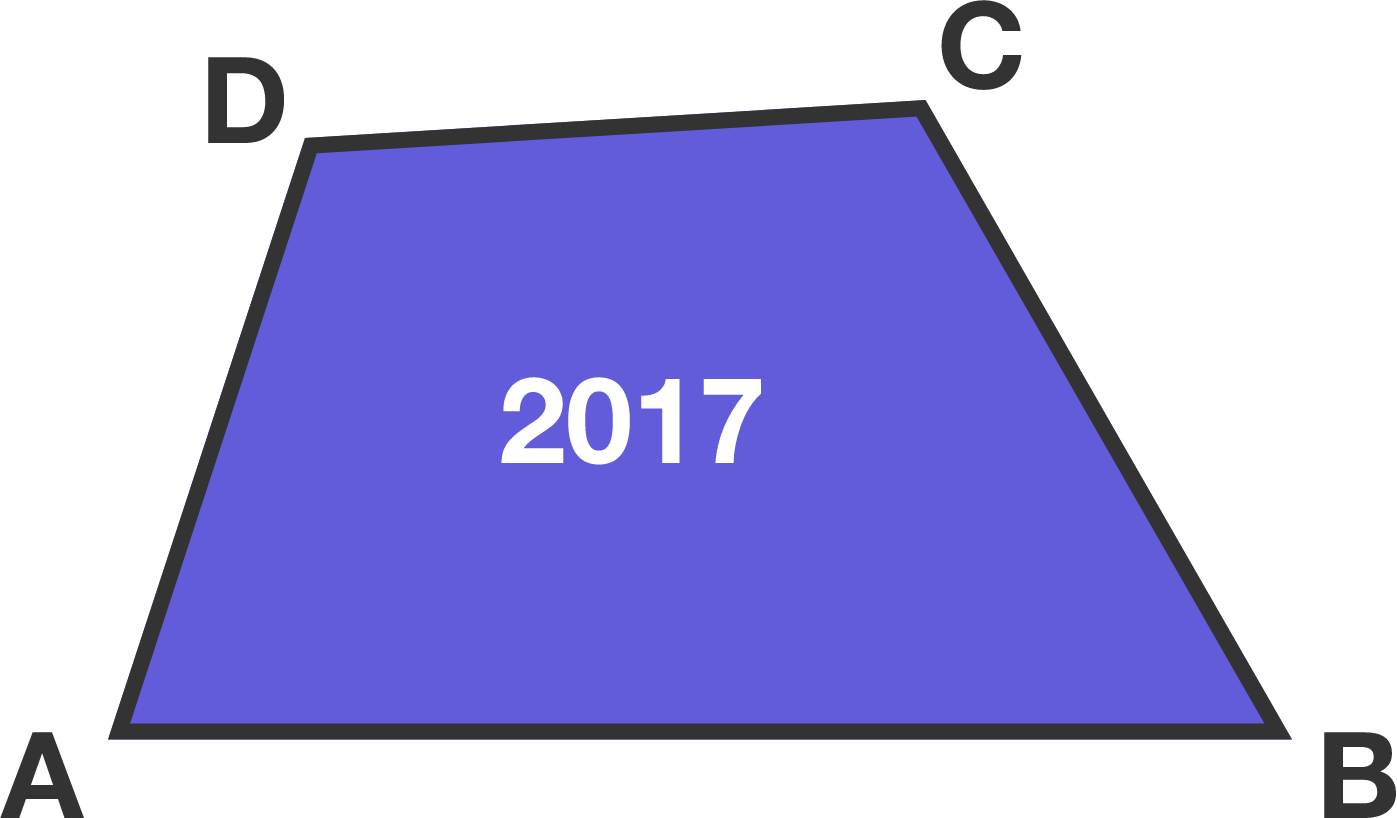# Minimum sum

Geometry Level 3

The area of the convex quadrilateral $ABCD$ is $2017$.What is the minimum value of $AB \times CD + AD \times BC?$

×